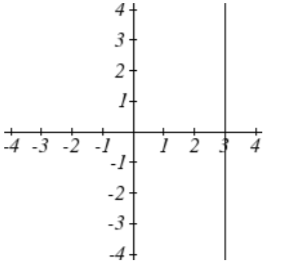$$\newcommand{\id}{\mathrm{id}}$$ $$\newcommand{\Span}{\mathrm{span}}$$ $$\newcommand{\kernel}{\mathrm{null}\,}$$ $$\newcommand{\range}{\mathrm{range}\,}$$ $$\newcommand{\RealPart}{\mathrm{Re}}$$ $$\newcommand{\ImaginaryPart}{\mathrm{Im}}$$ $$\newcommand{\Argument}{\mathrm{Arg}}$$ $$\newcommand{\norm}{\| #1 \|}$$ $$\newcommand{\inner}{\langle #1, #2 \rangle}$$ $$\newcommand{\Span}{\mathrm{span}}$$

# 2.2.2E: Graphs of Linear Functions (Exercises)

$$\newcommand{\vecs}{\overset { \rightharpoonup} {\mathbf{#1}} }$$ $$\newcommand{\vecd}{\overset{-\!-\!\rightharpoonup}{\vphantom{a}\smash {#1}}}$$$$\newcommand{\id}{\mathrm{id}}$$ $$\newcommand{\Span}{\mathrm{span}}$$ $$\newcommand{\kernel}{\mathrm{null}\,}$$ $$\newcommand{\range}{\mathrm{range}\,}$$ $$\newcommand{\RealPart}{\mathrm{Re}}$$ $$\newcommand{\ImaginaryPart}{\mathrm{Im}}$$ $$\newcommand{\Argument}{\mathrm{Arg}}$$ $$\newcommand{\norm}{\| #1 \|}$$ $$\newcommand{\inner}{\langle #1, #2 \rangle}$$ $$\newcommand{\Span}{\mathrm{span}}$$ $$\newcommand{\id}{\mathrm{id}}$$ $$\newcommand{\Span}{\mathrm{span}}$$ $$\newcommand{\kernel}{\mathrm{null}\,}$$ $$\newcommand{\range}{\mathrm{range}\,}$$ $$\newcommand{\RealPart}{\mathrm{Re}}$$ $$\newcommand{\ImaginaryPart}{\mathrm{Im}}$$ $$\newcommand{\Argument}{\mathrm{Arg}}$$ $$\newcommand{\norm}{\| #1 \|}$$ $$\newcommand{\inner}{\langle #1, #2 \rangle}$$ $$\newcommand{\Span}{\mathrm{span}}$$

Section 2.2 exercise

Match each linear equation with its graph1. $$f(x)=-x-1$$

2. $$f(x)=-2x-1$$

3. $$f(x)=-\dfrac{1}{2} x-1$$

4. $$f(x)=2$$

5. $$f(x)=2+x$$

6. $$f(x)=3x+2$$

Sketch a line with the given features

7. An x-intercept of (-4, 0) and y-intercept of (0, -2)

8. An x-intercept of (-2, 0) and y-intercept of (0, 4)

9. A vertical intercept of (0, 7) and slope $$-\dfrac{3}{2}$$

10. A vertical intercept of (0, 3) and slope $$\dfrac{2}{5}$$

11. Passing through the points (-6,-2) and (6,-6)

12. Passing through the points (-3,-4) and (3,0)

Sketch the graph of each equation

13. $$f(x)=-2x-1$$

14. $$g(x)=-3x+2$$

15. $$h(x)=\dfrac{1}{3} x+2$$

16. $$k(x)=\dfrac{2}{3} x-3$$

17. $$k(t)=3+2t$$

18. $$p(t)=-2+3t$$

19. $$x=3$$

20. $$x=-2$$

21. $$r(x)=4$$

22. $$q(x)=3$$

23. If $$g(x)$$ is the transformation of $$f(x)=x$$ after a vertical compression by $$3/4$$, a shift left by 2, and a shift down by 4

a. Write an equation for $$g(x)$$
b. What is the slope of this line?
c. Find the vertical intercept of this line.

24. If $$g(x)$$ is the transformation of $$f(x)=x$$ after a vertical compression by $$1/3$$, a shift right by 1, and a shift up by 3

a. Write an equation for $$g\left(x\right)$$
b. What is the slope of this line?
c. Find the vertical intercept of this line.

Write the equation of the line shown

25.26.27.28.Find the horizontal and vertical intercepts of each equation

29. $$f(x)=-x+2$$

30. $$g(x)=2x+4$$

31. $$h(x)=3x-5$$

32. $$k(x)=-5x+1$$

33. $$-2x+5y=20$$

34. $$7x+2y=56$$

Given below are descriptions of two lines. Find the slopes of Line 1 and Line 2. Is each pair of lines parallel, perpendicular or neither?

35. Line 1: Passes through $$(0,6)$$ and $$(3,-24)$$
Line 2: Passes through $$(-1,19)$$ and $$(8,-71)$$

36. Line 1: Passes through $$(-8, -55)$$ and $$(10, 89)$$
Line 2: Passes through $$(9, -44)$$ and $$(4,-14)$$

37. Line 1: Passes through $$(2,3)$$ and $$(4,-1)$$
Line 2: Passes through $$(6,3)$$ and $$(8,5)$$

38. Line 1: Passes through $$(1, 7)$$ and $$(5,5)$$
Line 2: Passes through $$(-1,-3)$$ and $$(1,1)$$

39. Line 1: Passes through $$(0, 5)$$ and $$(3,3)$$
Line 2: Passes through $$(1,-5)$$ and $$(3,-2)$$

40. Line 1: Passes through $$(2,5)$$ and $$(5,-1)$$
Line 2: Passes through $$(-3,7)$$ and $$(3,-5)$$

41. Write an equation for a line parallel to $$f(x)=-5x-3$$ and passing through the point (2,-12)

42. Write an equation for a line parallel to $$g(x)=3x-1$$ and passing through the point (4,9)

43. Write an equation for a line perpendicular to $$h(t)=-2t+4$$ and passing through the point (-4,-1)

44. Write an equation for a line perpendicular to $$p(t)=3t+4$$ and passing through the point (3,1)

45. Find the point at which the line $$f(x)=-2x-1$$ intersects the line $$g(x)=-x$$

46. Find the point at which the line $$f(x)=2x+5$$ intersects the line $$g(x)=-3x-5$$

47. Use algebra to find the point at which the line $$f(x)= -\dfrac{4}{5} x +\dfrac{274}{25}$$ intersects the line $$h(x)=\dfrac{9}{4} x +\dfrac{73}{10}$$

48. Use algebra to find the point at which the line $$f(x)=\dfrac{7}{4} x +\dfrac{457}{60}$$ intersects the line $$g(x)=\dfrac{4}{3} x +\dfrac{31}{5}$$

49. A car rental company offers two plans for renting a car.
Plan A: 30 dollars per day and 18 cents per mile
Plan B: 50 dollars per day with free unlimited mileage
How many miles would you need to drive for plan B to save you money?

50. You’re comparing two cell phone companies.
Company A: $20/month for unlimited talk and text, and$10/GB for data.
Company B: \$65/month for unlimited talk, text, and data.
Under what circumstances will company A save you money?

Find a formula for each piecewise defined function.

51.52.53. Sketch an accurate picture of the line having equation $$f(x)=2-\dfrac{1}{2} x$$. Let $$c$$ be an unknown constant. [UW]

a. Find the point of intersection between the line you have graphed and the line $$g(x)=1+cx$$; your answer will be a point in the $$xy$$ plane whose coordinates involve the unknown $$c$$.
b. Find $$c$$ so that the intersection point in (a) has $$x$$-coordinate 10.
c. Find $$c$$ so that the intersection point in (a) lies on the $$x$$-axis.

1. E

3. D

5. B

7.9.11.13.15.17.19.21.23. a. $$g(x) = \dfrac{3}{4} (x + 2) - 4$$
b. 3/4
c. -5/2

25. $$y = 3$$

27. $$x = -3$$

 Vertical Intercept Horizontal Intercept 29. (0, 2) (2, 0) 31. (0, -5) (5/3, 0) 33. (0, 4) (-10, 0)

35. Line 1: $$m = -10$$ Line 2: $$m = -10$$ Parallel

37. Line 1: $$m = -2$$ Line 2: $$m = 1$$ Neither

39. Line 1: $$m = -\dfrac{2}{3}$$ Line 2: $$m = \dfrac{3}{2}$$ Perpendicular

41. $$y = -5x - 2$$

43. $$y = \dfrac{1}{2} t + 1$$

45. (-1, 1)

47. (1.2, 10)

49. Plan B saves money if the miles are > 111$$\dfrac{1}{9}$$

51. $$f(x) = \begin{cases} 2x + 3 & if & -3 \le x < -1 \\ x - 1 & if & -1\le x \le 2 \\ -2 & if & 2 < x \le 5 \end{cases}$$